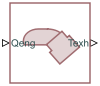# Exhaust Manifold Thermal

Thermodynamics of combustion engine exhaust manifold

Since R2022a

•Libraries:
Simscape / Driveline / Engines & Motors / Engine Subcomponents

## Description

The Exhaust Manifold Thermal block represents the abstracted thermodynamics of a combustion engine exhaust manifold. The block integrates with the SI Combustion Cylinder block.

### Equations

The block takes exhaust gas flow from the SI Combustion Cylinder blocks and computes the exhaust manifold temperature, Texh, which the block outputs at port Texh. The block calculates the output using a lumped-parameter model, such that

`${C}_{exh}{M}_{exh}\frac{d}{dt}{T}_{exh}={h}_{exh}-{h}_{x,}$`

where

• Cexh is the Heat capacity of exhaust wall parameter.

• Mexh is the Mass of exhaust wall parameter.

• hexh is the heat flow at the exhaust manifold.

• hx is the heat exchange between the exhaust gas and the environment.

The block calculates hx as

`${h}_{x}={S}_{exh}\left({h}_{convec}\left({T}_{exh}-{T}_{amb}\right)-{k}_{ra}\sigma \left({T}_{exh}^{4}-{T}_{amb}^{4}\right)\right),$`

where

• Sexh is the Surface area of exhaust wall parameter.

• hconvec is the Convective heat transfer coefficient for the outer side of exhaust parameter.

• kra is the Radiation constant parameter.

• σ is the Stefan-Boltzmann constant parameter.

• Tamb is the Ambient temperature parameter.

The block finds hexh as

`${k}_{gas}\frac{d}{dt}{h}_{exh}={h}_{in}-{h}_{exh},$`

where kgas is the Time constant of exhaust heat flow parameter, and hin is the instantaneous heat flow from the engine exhaust outlets to the exhaust manifold. The instantaneous heat flow is the sum of the individual instantaneous heat flows at the exhaust outlets, hi, such that

`${h}_{in}=\sum _{i=1}^{{N}_{ports}}{h}_{i},$`

where Nports is the Number of exhaust ports parameter.

## Ports

### Input

expand all

Physical signal input port associated with the gas energy flow, in W. Attach this port to the Weo physical signal output port on the SI Combustion Cylinder block.

### Output

expand all

Physical signal output port associated with the lumped-parameter exhaust manifold temperature, in K.

## Parameters

expand all

Number of SI Combustion Cylinder blocks.

Temperature of the air entering the intake.

Surface area of the exhaust wall. This is only the surface area of the internal wall that makes contact with the exhaust.

Mass of the exhaust wall.

Heat capacity of the exhaust wall.

Time constant for the rate of exhaust heat flow.

Convective heat transfer coefficient for the outer side of the exhaust.